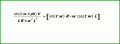# 2nd order differential equation

#### Menail

Joined Mar 25, 2021
4

#### crutschow

Joined Mar 14, 2008
32,859
Okay.
We don't do homework here, but we can help.
We're waiting for your attempt at a solution.

#### Menail

Joined Mar 25, 2021
4
This is a 1st order equation, not 2nd order.
okay ,kindly check the solution

#### MrAl

Joined Jun 17, 2014
10,593
This is just practice question and i tried that .Here i got stuckView attachment 233983View attachment 233984View attachment 233985
okay ,kindly check the solution
See attachment.

Are you saying that your solution is that in the attachment?
I simply retyped your solution from your last solution sheet but note some change in the order of some variables which does not change the implied equivalency.

Also, the presence of the open switch in the circuit brings into question whether or not we are supposed to solve for the exponential functions that occur when a switch closes in almost any circuit. For the time being, i will assume that we are looking for the sinusoidal steady state response which means we would only find sinusoidal terms in the solution. If this is true then you can also check your results by replacing the inductor with it's impedance and using complex math to solve for the amplitude and phase shift of the current. That idea provides you with a way to self check your time domain solution.

If that first paragraph above is so, then your solution can not be correct.
The reason is that for a linear network with a sinusoidal forcing function the solution will always come out to be a sinusoidal function with the SAME frequency as the driving function and with NO added frequencies. You can have sums and differences of sines and cosines and possibly some exponential functions, but you will never see a change in frequency unless the network has at least one nonlinear function in it and even then there is the possibility that the frequency will not change (possibly some added frequencies though).

To show this, set the constants (except for w) to some set value like 1 and also leave the time variable 't' alone.. So V=1, L=1,R=1, and you can set the phase 'ph' to zero or if you prefer some non zero constant.
Next, simply multiply everything out and simplify using trigonometric identities. What you end up with is at least one sinusoidal component that has twice the frequency. That's because when you multiply two sinusoidal functions you get a doubling of the frequency. For example,
sin(w*t)*sin(w*t)=1/2-cos(2*w*t)/2
and we can see that the angular frequency changed from "w" to "2*w" which is 2 times the original frequency. This kind of change never happens in a linear circuit with a pure sinusoidal input.

So check your work over again and see what you can discover.

This is your solution rewritten and you can see that we have at least one sin(wt)*sin(wt) product which causes a change in frequency so it can not be correct...Last edited:
•Menail